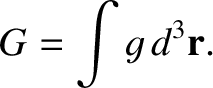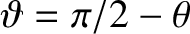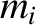Next: Plane Waves in a Up: Waves in Cold Plasmas Previous: Waves in Cold Plasmas

# Introduction

The cold-plasma equations describe waves, and other perturbations, which propagate through a plasma much faster than a typical thermal velocity. It is instructive to consider the relationship between the collective motions described by the cold-plasma model and the motions of individual particles that we studied in Sect. 2. The key observation is that in the cold-plasma model all particles (of a given species) at a given position effectively move with the same velocity. It follows that the fluid velocity is identical to the particle velocity, and is, therefore, governed by the same equations. However, the cold-plasma model goes beyond the single-particle description because it determines the electromagnetic fields self-consistently in terms of the charge and current densities generated by the motions of the constituent particles of the plasma.

What role, if any, does the geometry of the plasma equilibrium play in determining the properties of plasma waves? Clearly, geometry plays a key role for modes whose wave-lengths are comparable to the dimensions of the plasma. However, we shall show that modes whose wave-lengths are much smaller than the plasma dimensions have properties which are, in a local sense, independent of the geometry. Thus, the local properties of small-wave-length oscillations are universal in nature. To investigate these properties, we may, to a first approximation, represent the plasma as a homogeneous equilibrium (corresponding to the limit, whereis the magnitude of the wave-vector, andis the characteristic equilibrium length-scale).Next: Plane Waves in a Up: Waves in Cold Plasmas Previous: Waves in Cold Plasmas
Richard Fitzpatrick 2011-03-31